##1. Generalized isotomic transformation

This is another name of the generalized isogonal transformation defined by a system (ABC, K) consisting of a triangle and a point K not on the side-lines of ABC. The definition and the first properties of this transformation are discussed in IsogonalGeneralized.html . Since both well known transformations of Isotomy and Isogonality with respect to a triangle are special cases of such a general transformation we have the right to call it both generalized isogonal or/and generalized isotomic transformation.
In the following figure the basic facts for such a system (ABC,K) are discussed. The properties result from corresponding properties that are valid and trivial to show for equilateral triangles. The figure results by mapping the system (A0B0C0, O) of an equilateral and its centroid to the system (ABC,K) of an arbitrary triangle and an arbitrary point K. The map used for this is the uniquely defined projectivity F mapping points {A0,B0,C0,O} correspondingly to {A,B,C,K}. Every property of the figure listed below results by applying F to the corresponding figure for the equilateral discussed in section 10 of the aforementioned reference.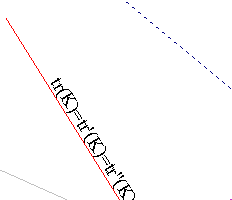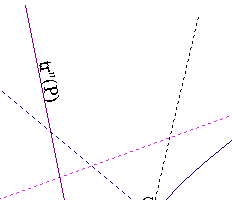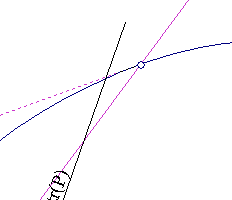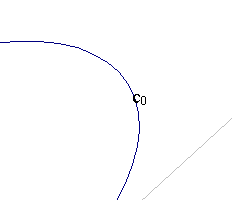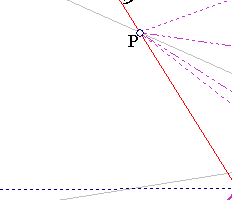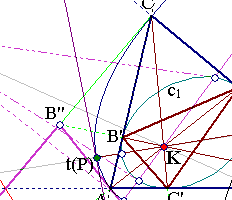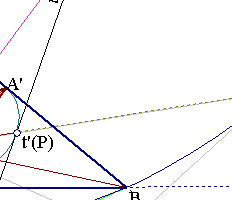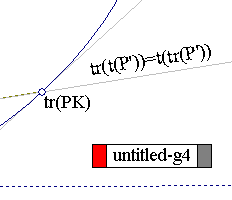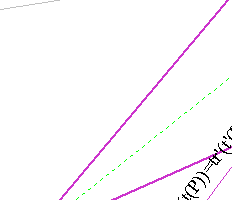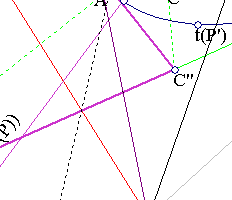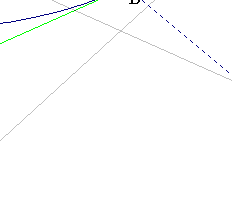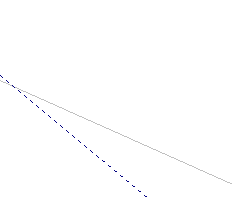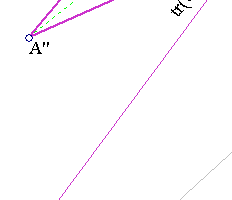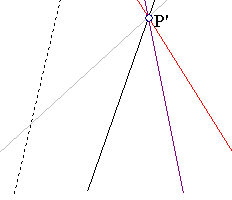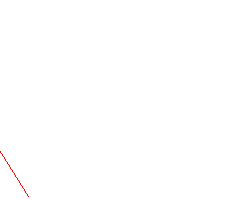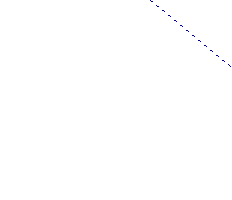ABC denotes the triangle of reference. A'B'C' the triangle of traces of point K, and A''B''C'' the triangle of harmonic conjugates of K. Generalized isotomy with respect to the systems (ABC,K), (A'B'C',K), (A''B''C'',K) is denoted correspondingly by t, t', t''. Trilinear polars/poles with respect to these systems are denoted by tr, tr', tr''.
 The trilinear polar of K with respect to any of these systems is the same line PP' (PP'=tr(K)=tr'(K)=tr''(K)).
 The tripoles tr(PK) of lines through K generate a circumconic c0 of ABC. Analogously tr'(K) and tr''(K) generate conics c1, c2 circumscribing A'B'C' and A''B''C''. Conic c1 is the dual of c0.
 Line tr(K) is the polar of K with respect to any one of these conics.
 For every point P on tr(K) points {t(P),t'(P),t''(P)} are on the line passing through K and tr(PK).
 For every point P on tr(K) lines {tr(P),tr'(P)} are tangents correspondingly to {c1,c0} at {t'(P),t(P)} and meet at a point P' lying on tr(K). Point P' is the pole of the line through {t(P), t'(P)} with respect to both conics c0 and c1.
 For every point P on tr(K) lines tr(t(P)) and tr(t'(P)) coincide with a line through K, which is the common polar of P with respect to both conics {c0,c1}.

##2. The sequence of triangles/conics

 Starting from triangle A'B'C' and taking successive harmonic associates of K one creates triangles ABC, A''B''C'' and, proceeding in the same vein, a sequence S of triangles t(n), each having to its predecessor the relation that ABC has to A'B'C'.
Sequence S can be extended also inside A'B'C' by taking successive traces with respect to K. The trilinear polar of K with respect to every triangle of this sequence is the same line. This extends [1.2] for the whole of the sequence S.
 Denote by trn the operation of taking the tripolar/tripole with respect to a triangle t(n) of the previous sequence S. Fixing such a triangle t(n) and taking the tripoles of trn(PK) of lines through K generates a conic cn circumscribing t(n). This produces a sequence of conics Sc belonging to the family of conics generated by two members of Sc. The trilinear polar tr(K) belongs to this family as a double line (degenerate conic).
 Since conics cn belong to a family of conics they are disjoint. Line tr(K) is the polar of K with respect to any conic cn of the family. This generalizes [1.4].
 Also [1.5-1.7] generalize in the following way. Denote bei tn the (generalized) isogonal conjugation with respect to triangle t(n). For every point P on tr(K) there is another point P' on the same line with the property: For every n points
{tn(P),trn(PK)} are the intersection points of conic cn with the polar of P'. The polars of points P on tr(K) are the same for every conic member of Sc therefore one can say for such a point P' the polar of P' leaving undeterminate the conic of reference. Further for every point P on tr(K) line trn(tn(P)) coincides with the polar of P.

##3. The homography viewpoint

The system (ABC,K) of a triangle and a point uniquely defines a homography F (perspectivity) fixing K and mapping {A,B,C} to the traces {A',B',C'} of K.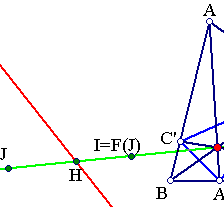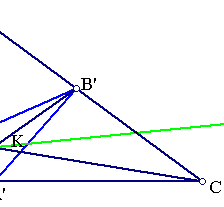This homography is conjugate F=G*F0*G-1 to the antihomothety F0 of the equilateral to its medial triangle. G is the projectivity mapping the system (A0B0C0,O) of the equilateral triangle and its center to (ABC, K). It follows immediately that F is a homology (see Perspectivity.html ) with coefficient k=-2 having one isolated fixed point K and a line consisting entirely of fixed points which is no other than the trilinear polar tr(K) with respect to ABC.
The various triangles of the sequence S of section 2 are simply the result of repeated application of F or F-1 i.e. t(n) = Fn(ABC).
Analogously the sequence of conics is created by applying repeatedly F or F-1 to the circumconic c0.
Remark The fact that the homology coefficient is equal to -2 implies that for every point J points {J, I=F(J), K} are collinear and if H is the intersection point of their line with tr(K), then the cross ratio (J,I,K,H) = -2.
See the file TrilinearProjectivity.html for a figure displaying part of the sequence of triangles and the sequence of conics.